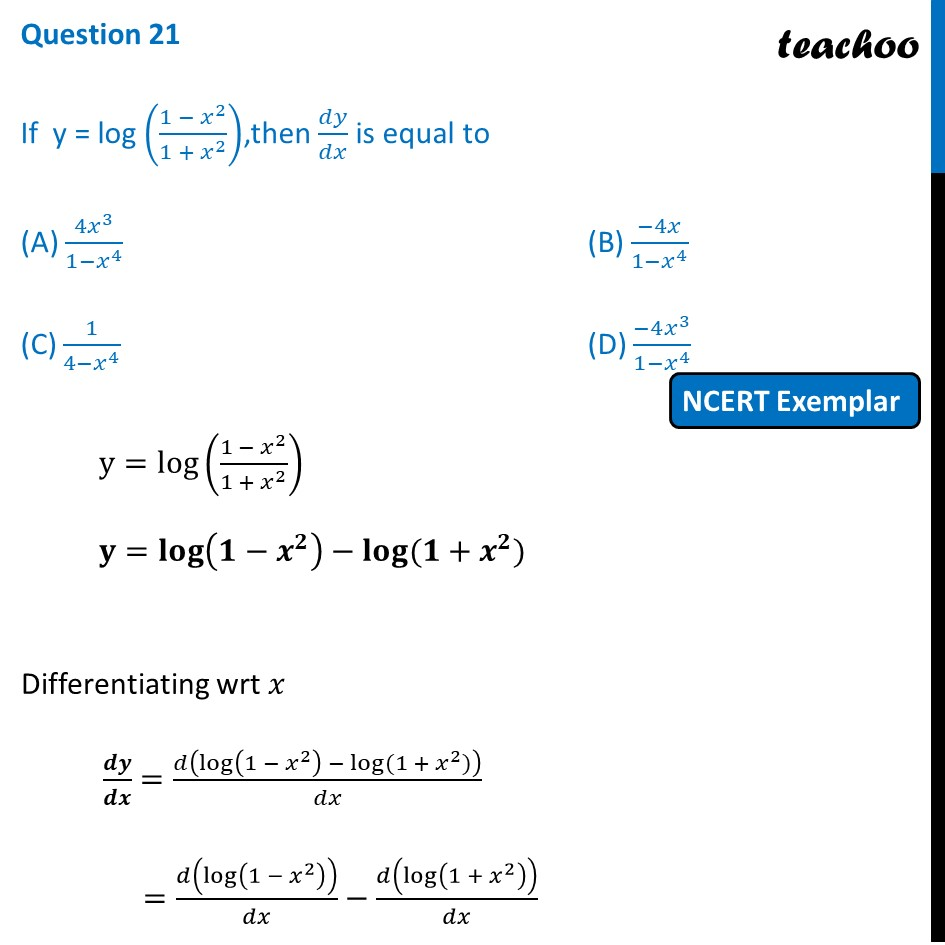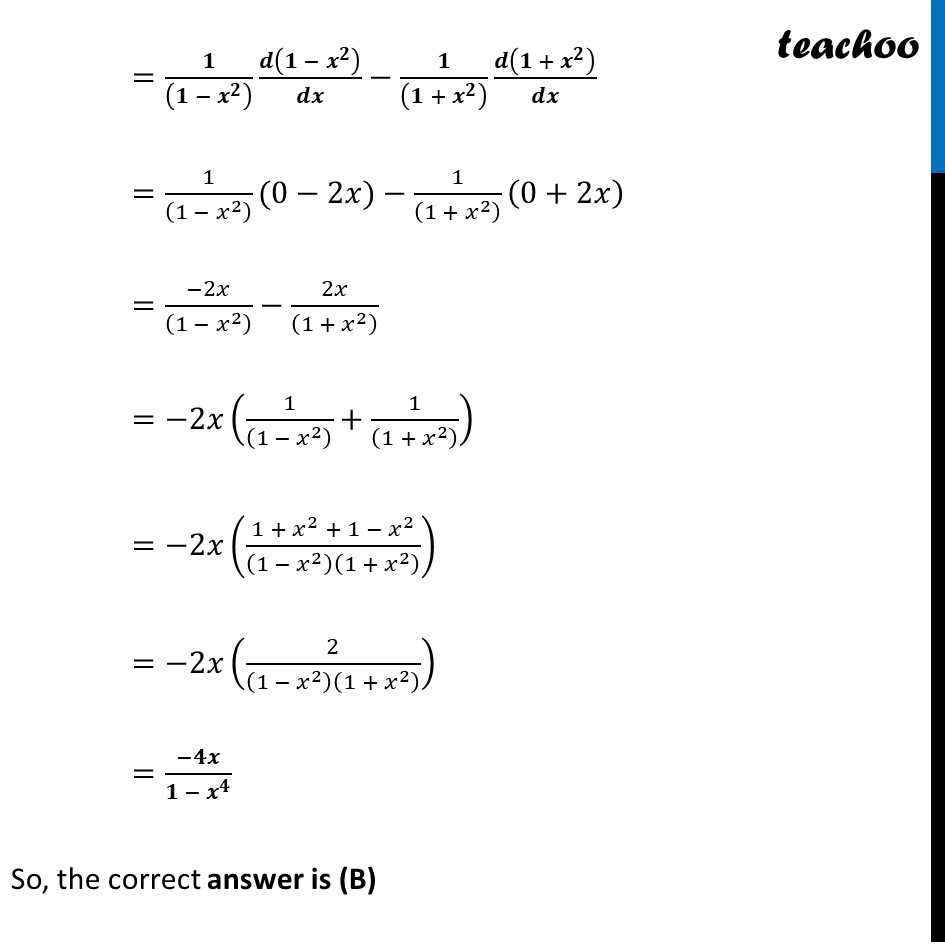NCERT Exemplar - MCQs

Chapter 5 Class 12 Continuity and Differentiability
Serial order wise

## (D) (-4x 3 )/(1-x 4 )Learn in your speed, with individual attention - Teachoo Maths 1-on-1 Class

### Transcript

Question 19 If y = log ((1 − 𝑥^2)/(1 + 𝑥^2 )),then 𝑑𝑦/𝑑𝑥 is equal to (A) 〖4𝑥〗^3/(1−𝑥^4 ) (B) (−4𝑥)/(1−𝑥^4 ) (C) 1/(4−𝑥^4 ) (D) (−4𝑥^3)/(1−𝑥^4 ) y=log((1 − 𝑥^2)/(1 +〖 𝑥〗^2 )) 𝐲=𝐥𝐨𝐠(𝟏−𝒙^𝟐 )−𝐥𝐨𝐠⁡〖(𝟏+𝒙^𝟐)〗 Differentiating wrt 𝑥 𝒅𝒚/𝒅𝒙=𝑑(log(1 − 𝑥^2 ) − log⁡〖(1 + 𝑥^2)〗 )/𝑑𝑥 =𝑑(log(1 − 𝑥^2 ))/𝑑𝑥−𝑑(log(1 + 𝑥^2 ))/𝑑𝑥 =𝟏/((𝟏 − 𝒙^𝟐 ) ) 𝒅(𝟏 − 𝒙^𝟐 )/𝒅𝒙−𝟏/((𝟏 + 𝒙^𝟐 ) ) 𝒅(𝟏 + 𝒙^𝟐 )/𝒅𝒙 =1/((1 − 𝑥^2 ) )(0−2𝑥)−1/((1 + 𝑥^2 ) ) (0+2𝑥) =(−2𝑥)/((1 − 𝑥^2 ) )−2𝑥/((1 + 𝑥^2 ) ) =−2𝑥(1/((1 −〖 𝑥〗^2 ) )+1/((1 +〖 𝑥〗^2 ) )) =−2𝑥((1 + 𝑥^2 + 1 −〖 𝑥〗^2)/(1 − 𝑥^2 )(1 + 𝑥^2 ) ) =−2𝑥(2/(1 −〖 𝑥〗^2 )(1 + 𝑥^2 ) ) =(−𝟒𝒙)/(𝟏 − 𝒙^𝟒 ) So, the correct answer is (B)